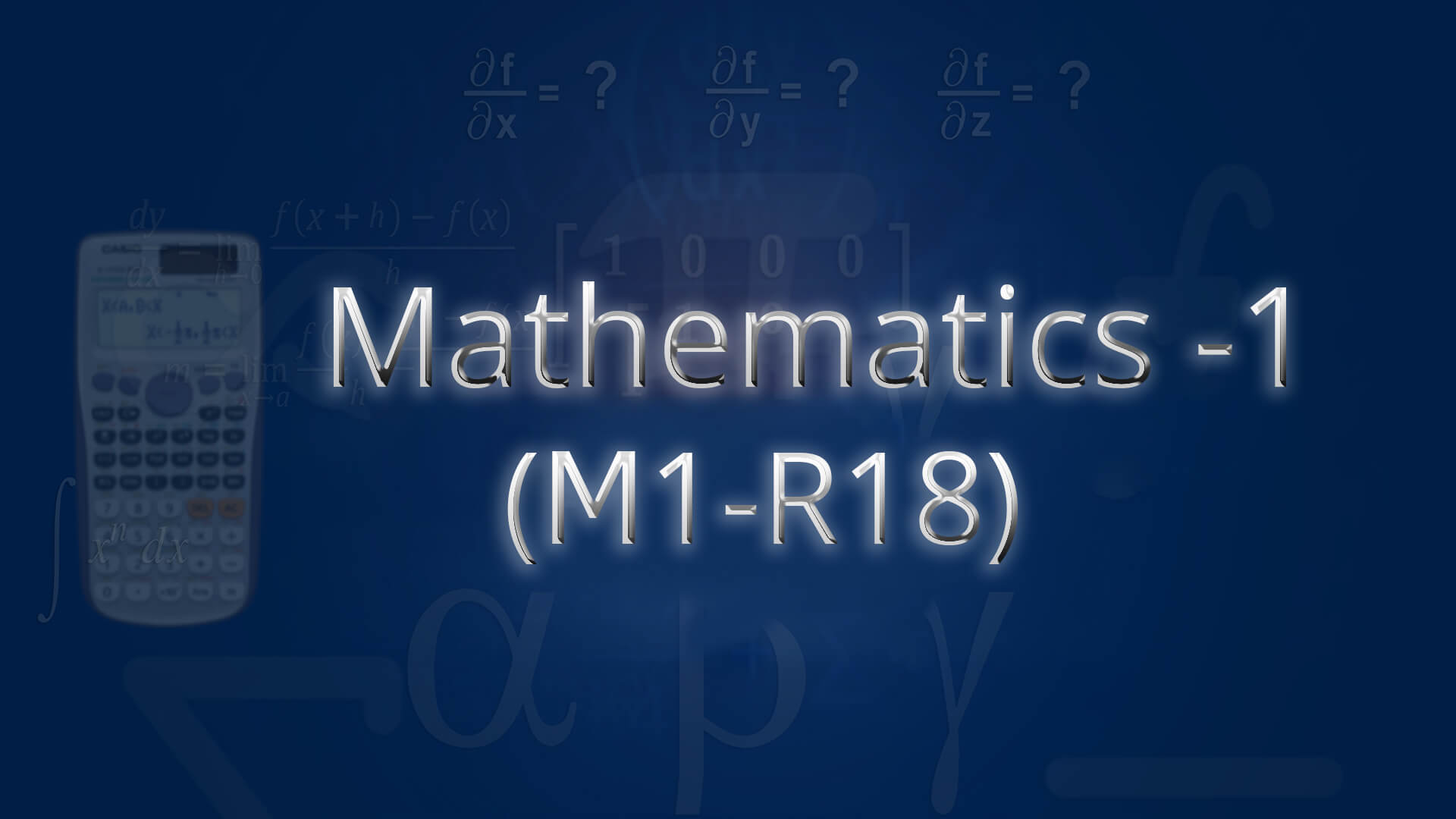## Mathematics 1(M1-R18) by Mr C Srinivas MSc ,BEd (Osmania) The course is designed to equip the students with the necessary mathematical skills and techniques that are essential for an engineering course. The skills derived from the course will help the student from a necessary base to develop analytic and design concepts. Code : M1B.Tech1 Recommended For : B.Tech 1st Year JNTU,OU

#### After Learning this subject,you should be able to

• After going through this course , a student will be able to confidently appear M1 Examination with learning in the following areas
• Write the matrix representation of a set of linear equations and solutions
• Find Eigen values and vectors
• Reduce the quadratic form canonical orthogonal transformations
• Analyse the nature of sequence series
• Solve the applications on the mean value theorems
• Find extreme values of functions of two variables with or without constraints

## Curriculum 10:28:55   units   |   5

• 2:43:20

UNIT 1
Matrices: Types of real matrices and complex matrices, Types of real matrices and complex matrices Part 1, Types of real matrices and complex matrices Part 2, Types of real matrices and complex matrices Part 3, Rank Of Matrix, Rank Of Matrix Problem, Echelonform Problem Part 1, Echelonform Problem Part 2, Normal Form Or Canonical Form, Normalform (or) Canonical form problem, Inverse of Non-Singular matrices by Gauss-Jordan Method, Solution of system of Linear Equations, Solution of system of Homogeneous Linear Equations, Consistency and solution of linear systems(Homogeneous and Non-Homogeneous) Part 1, Consistency and solution of linear systems(Homogeneous and Non-Homogeneous) Part 2, Gauss Seidel iteration method, Gauss Elimination and Seidel iteration method Part 1, Gauss Elimination and Seidel iteration method Part 2

• 2:40:38

UNIT 2
Eigen Values and Eigen Vectors (Linear Transformation and Orthogonal transformation): Eigen Values and Eigen Vectors, Eigen Values and Eigen Vectors and their properties Theorem, Eigen Values and Eigen Vectors and their properties Theorem Part 1, Eigen Values and Eigen Vectors Problems Part 1, Eigen Values and Eigen Vectors Problems Part 2, Eigen Values and Eigen Vectors Problems Part 3, Diagonalization of matrix, Diagonalization of a matric by Othogonal Reduction, Diagonalization of matrix (Problem 1), Diagonalization of matrix (Problem 2), Cayley-Hamilton Theorem(Without Proof), Cayley-Hamilton Theorem Problem, Finding Inverse and power of a matrix by Cayley-Hamilton Theorem, Quadratic Forms and Nature Of The Quadratic Form, Quadratic Forms and Nature of the Quadratic forms part 1, Quadratic Forms and Nature of the Quadratic forms part 2, Reduction of Quadratic Form To Canonical Form, Reduction of Quadratic form to canonical form by orthogonal transformation part-1, Reduction of Quadratic form to canonical form by orthogonal transformation part-2

• 1:19:16

UNIT 3
Sequences and Series: Definition of Sequence,Limit,Convergent,Divergent and Oscillatory sequences, Convergent,Divergent and Oscillatory series Part 1, Convergent,Divergent and Oscillatory series Part 2, Limit Comparison Test, Limit Comparison Test Problem 1, Limit Comparison Test Problem 2, Limit Comparison Test Problem 3, p-test,D-Alembert's ratio test, Raabe's test, Cauchy's nth Root Test & Cauchy's Integral Test, Cauchy's Integral test and Cauchy's root test Problem 1, Cauchy's Integral test and Cauchy's root test Problem 2, Cauchy's Integral test and Cauchy's root test Problem 3, Logarithmic test, Alternating series : Leibnitz test, Alternating series : Leibnitz test Problem, Alternating Convergent series : Absolute and Conditionally convergence

• 1:40:40

UNIT 4
Calculus: Rolle's Theorem, Geometrical Interpretation Of Rolle's Theorem, Mean Value Theorems:Rolle's Theorem Part 1, Mean Value Theorems:Rolle's Theorem Part 2, Mean Value Theorems:Rolle's Theorem Part 3, Lagrange's Mean Value Theorem, Geometrical Interpretation Of Lagrange's Mean Value Theorem, Lagrange's Mean value theorem and applications Part 1, Lagrange's Mean value theorem and applications Part 2, Cauchy's Mean Value Theorem, Taylor's Series, Surface areas and volumes of solid of revolution, Applications of definite integrals to evaluate surface areas and volumes of revolution of curves, Definition of improper integral, Beta Gamma Function and applications Part 1, Beta Gamma Function and applications Part 2, Beta Gamma Function and applications Part 3, Beta Gamma Function and applications Part 4, Beta Gamma Function and applications Part 5, Beta Gamma Function and applications Part 6

• 2:05:01

UNIT 5
Multivariable Calculus(Partial Differentiation and Applications): Euler's Theorem, Euler's Theorem Problem 1, Euler's Theorem Problem 2, Total Derivative, Chain Rule of Partial Derivation, Chain Rule of Partial Derivation Problem 1, Chain Rule of Partial Derivation Problem 2, Chain Rule of Partial Derivation Problem 3, Jacobian Determinant, Jacobian Problem 1, Jacobian Problem 2, Functional Dependence and Independence, Maxima and minima of functions of 1 & 2 variables, Lagranges Method, Max and min of functions of 2 & 3 variables using method of Lagrange Multipliers Part 1, Max and min of functions of 2 & 3 variables using method of Lagrange Multipliers Part 2, Max and min of functions of 2 & 3 variables using method of Lagrange Multipliers Part 3, Taylor's Series and Maclaurin's Series, Taylor's Series and Maclaurin's Series Part 1, Taylor's Series and Maclaurin's Series Part 2